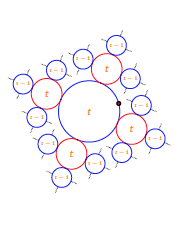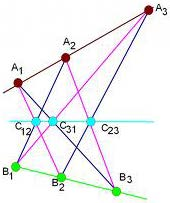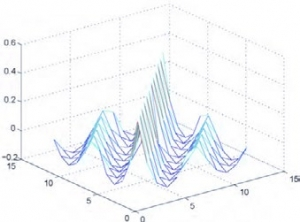## Research faculty members in this area

#### Arithmetic geometry, modular forms and number theory

Fermat’s last theorem says that there are no solutions to the equation x+ y= zwith x, y, n, z integers and n>2; its proof was completed by Andrew Wiles more than 350 years after the problem first appeared. The significance of the proof, however, went far beyond settling this long-standing conjecture: it illustrated a profound connection between the arithmetic of certain geometric objects (elliptic curves) and objects from number theory (automorphic forms). Relations of this spirit are at the heart of many of the key questions and conjectures in modern number theory, including the Birch–Swinnerton-Dyer conjectures and their generalizations and the Langlands programme.

Shimura varieties are geometric objects that encode a wealth of number-theoretic information; they form a bridge between the arithmetic-geometric and automorphic worlds and are therefore particularly useful in understanding the links between these two domains. Of particular interest in the department is a broad system of conjectures, at present still quite mysterious, relating arithmetic cycles on some Shimura varieties to the Fourier coefficients of automorphic forms.#### Commutative algebra

Hosting many intriguing problems with wide-ranging applications such as coding theory, computer science, statistics, and physics, Commutative Algebra is a riveting field to work in. Of particular interest in Commutative Algebra is the study of algebraic structures via numerical invariants of objects living in geometric and combinatorial settings. The rich overlap between Commutative Algebra, Combinatorics, and Geometry empowers us to solve problems using different viewpoints.

A simple example of the intersection of Algebra and Geometry is the use of equations to describe lines in the plane. Deep geometric information is encoded in numeric invariants of point sets. For example, an invariant called the Hilbert function which is a sequence of vector space dimensions can be used to determine configuration data about the point set (such as guaranteeing situations when some points lie on a line).

Similarly, there is an overlap between Algebra and Graph Theory. A graph is a collection of nodes with edges connecting the nodes. We assign to each node a variable and to each edge, a product of variables called a monomial which leads to the study of monomial ideals. In this direction, one uses properties of the graph such as the chromatic number to bound or describe algebraic information about the ideal.

#### Computer algebra

Computer Algebra is not Computer plus Algebra.

Pappus’ Theorem: Suppose A1, A2, A3 are collinear and so are B1, B2, B3. Then C12, C31, C23 are also collinear.

Computer algebra (also called symbolic computation) is a relatively recent research area in computer science and mathematics, where the mathematical computation is symbolic rather than numeric. Although numerical computations provide efficient solutions in many practical questions, computer algebra strives to address the different and arguably more difficult problems by providing exact solutions, or solutions parameterized by variables in the input. Another important component of computer algebra is to find/prove mathematical theorems and formulas.

In the past few decades, this method has developed very quickly and successfully been applied to many areas, for example, cryptography, control theory, physics, signal process, etc.

This theorem can be proved by computer algebra techniques! Sudoku can also be solved using computer algebra.#### Linear algebra and matrix theory

Because of its flexibility, utility and well-developed theory, linear algebra is a tool that is used ubiquitously throughout science and engineering. The theory of matrices all of whose entries are nonnegative is particularly rich and can be approached from analytic, combinatorial and computational perspectives. Further, nonnegative matrices and their variants appear in a number of contexts including demographic models, electrical networks and stochastic processes. Thus, nonnegative matrix theory not only enjoys a wide variety of motivating problems but also offers several avenues of entry for mathematical exploration. The Department has interest and expertise in developing nonnegative matrix theory in the analytical/combinatorial/computational directions, and in applying the results to problems in population dynamics, Markov chains, and spectral graph theory.#### Geometric group theory

Group theory began with the study of symmetries, that is, rigid motions of geometric objects, and has today become a flourishing field of abstract mathematics that brings together tools from algebra, combinatorics, topology, and analysis (to name a few).  In particular, one of the most exciting developments of the last few decades has been an approach to abstract algebraic questions that leans heavily on geometric intuition and topology, what has now become known as geometric group theory.  This relatively new field of pure mathematics studies groups via their actions on topological spaces, and via the geometry of their Cayley graphs, and has proved essential in resolving several longstanding open questions both in the field of algebra, and the field of topology (e.g. the Hannah Neumann conjecture, or the virtual first Betti number conjecture).